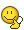# What is this function ?

## Recommended Posts

Dear All,

I'm trying to find function J(x) shown in attached file. Could somebody help me ? It seems that I don't have the right tool to solve itThanks and Regards.

Série.pdf

##### Share on other sites

That capitol Sigma is a summation of the function of P for all values of P between 0 and infinity. Not sure how to solve it though - I haven't done that for years. I'm sure someone else can point you in the right direction - there are some good maths guys here.##### Share on other sites

Not sure how to solve it though

It is an infinite loop.

public static void function(int x){
int p = 0;
while(true){
if(p%2 == 0}{
} else{
}
}

}

public static int factorial(int n) {
int result = 1;
for (int i = 1; i <= n; i++) {
result = result * i;
}
return result;
}

##### Share on other sites

It is an infinite loop.

It is the sum of an infinite series, which is not the same thing. Your program can never return the value of the function but it is often possible to calculate the value.

##### Share on other sites

You seem to have missed modifying p. And, I'd recommend decreasing the repeated code.

Also, the code will "explode" when the ints start overflowing. It won't be infinite!

Points for a non-recursive factorial. However, since we know (well, I'm assuming) p increases by 1 each time, we'd be able to keep the last factorial calculated and just multiply by the new p each time, rather than re-calculating all of 1 to p each time. Of course that makes it a non-generic factorial function, more of a "nextvalue" function. (I didn't make this change).

(Disclaimer: I didn't read the PDF, I'm just reacting to the code (and I don't "do" java).)

public static void function(int x){
int temp = 0;
int p = 0;
while(true){
temp = java.lang.Math.pow((x/2),​2p)/java.lang.Math.pow(factorial(p),2);
if(p%2 == 0}{
} else{
}
p++; // p was not changing
}
}

public static int factorial(int n) {
int result = 1;
for (int i = 1; i <= n; i++) {
result = result * i;
}
return result;
}


Edited by pzkpfw
##### Share on other sites

Dear All,

Thank you for your respective contributions. I do appreciate ! the reason why I need this function is because I need to integrate it in function of x.

Have your codes been typed on Matlab ? Do I need module "Symbolic Math Toolbox" to try your code ?

Thanks again and Regards,

Cédric

## Create an account

Register a new account